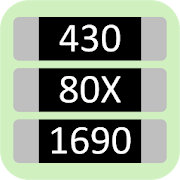# SMD Resistor Code - Calculator

## A simple to use SMD-code calculator for SMD resistors!

4.58 TOOLS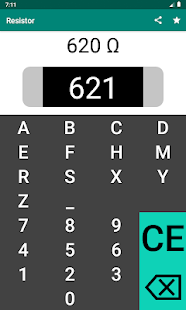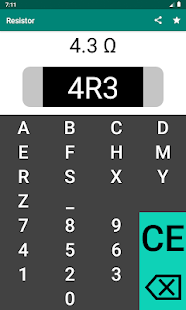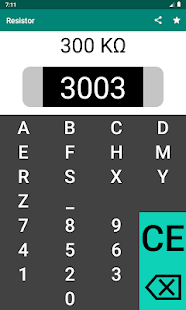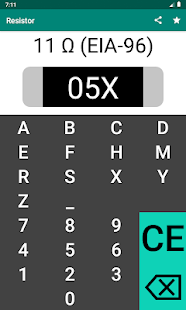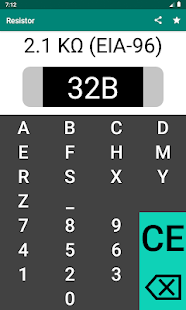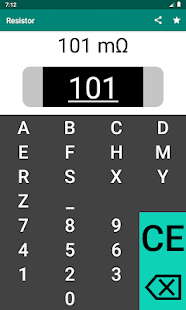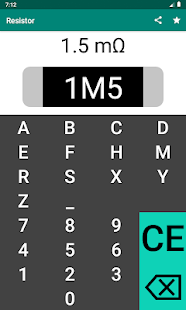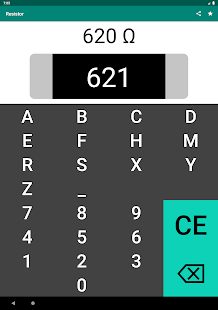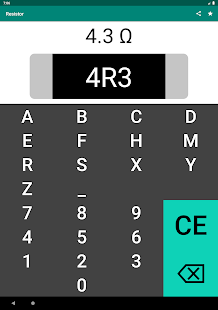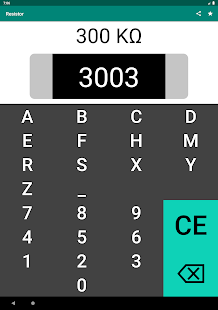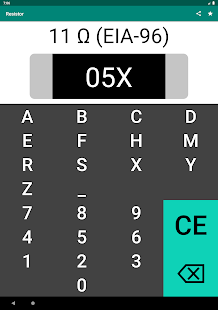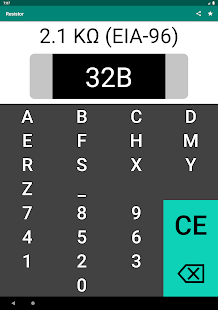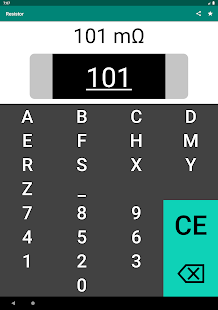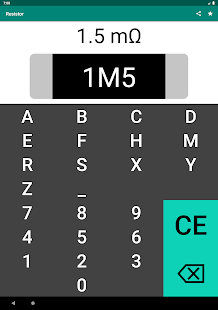The application is an easy-to-use SMD-code calculator for calculating the resistance of a resistor. The application supports the 3-digits, 4-digits, and EIA-96 coding systems .

Resistor
A resistor is a component that is used in electrical circuits to limit the current flow. The resistance of a resistor is measured in Ohms (Ω). When a current (I) of one Ampere passes through a resistor with a voltage drop (U) of one Volt, the resistance of a resistor (R) corresponds with one Ohm. This ratio is represented by Ohm's law: R = U ÷ I.

SMD-code
The codes on the SMD resistors identifies the resistance of a resistor. There are multiple coding systems that determines the resistance of a resistor: 3-digits, 4-digits, and EIA-96. In the following, the meaning of each coding system is described.

3-digits
In the 3-digit coding system, the first two numbers indicate the significant digits, where the third digit specifies the multiplier. The multiplier indicates how many zeros must be added to the two significant digits. In case the resistance is smaller than 10 Ohms, the letter R is used to indicate the position of the decimal point. In the following, a few examples are shown.

340 = 34 Ω
781 = 780 Ω
202 = 2000 Ω or 2 KΩ
5R5 = 5.5 Ω

4 digits
The 4-digit coding system is very similar to the 3-digit coding system. In the 4-digit coding system, the first three digits indicate the significant digits, where the fourth digit specifies the multiplier. The multiplier indicates how many zeros must be added to the three significant digits. In case the resistance is smaller than 100 Ohms, the letter R is used to indicate the position of the decimal point. In the following, a few examples are shown.

9100 = 910 Ω
2204 = 2.2 MΩ
0R10 = 0.1 Ω

EIA-96
The EIA-96 coding system consists of three characters. The first two characters are digits, which corresponds to the 3 significant digits of the resistance according a look-up table. The third character is a letter that indicates the multiplication factor of the resistance. In the following, a few examples are shown.

40A = 255 Ω
12E = 1.3 MΩ
52F = 34 MΩ

Small bug fixes.

### Details and Recent Ratings

Size: 4 MB
Version: 1.0 by Tom Hogenkamp
Updated: 22 August 2020 (402 days ago)
Released: 15 July 2020
Installations: more than 10 000
5 Stars: 318
4 Stars: 31
3 Stars: 19
2 Stars: 11
1 Star: 15

### Similar apps

Android Game Categories
Android App Categories